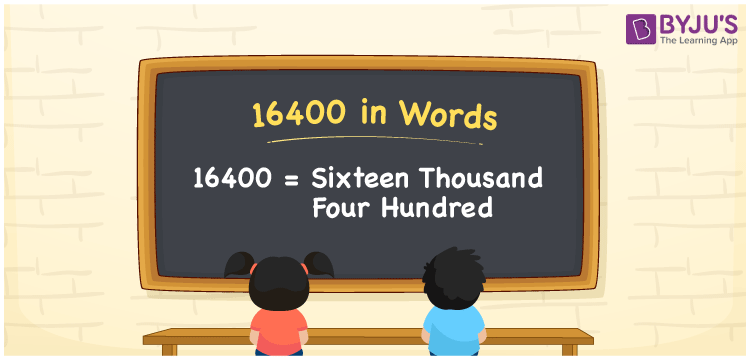# 16400 in Words

We can express 16400 in words as Sixteen Thousand Four Hundred. If an article on sports in a newspaper contains 16400 words, then we can say, “An article on sports in a newspaper contains Sixteen Thousand Four Hundred words”. 16400 is a cardinal number as it denotes a certain amount. Let us learn how to write 16400 in word form using a place value chart in this article.

 16400 in words Sixteen Thousand Four Hundred Sixteen Thousand Four Hundred in numerical form 16400

## 16400 in English Words

Generally, In Maths, we can write numbers in words using the English alphabet. Hence, we can read 16400 in English as Sixteen Thousand Four Hundred.## How to Write 16400 in Words?

Here, we will learn the method of writing the number 16400 in word form with the help of a place value chart of 5 columns since 16400 has five digits.

 Ten-Thousands Thousands Hundreds Tens Ones 1 6 4 0 0

Thus, the expanded form can be written as:

1 x Ten Thousand + 6 x Thousand + 4 x Hundred + 0 × Ten + 0 × One

= 1 x 10000 + 6 x 1000 + 4 x 100 + 0 x 10 + 0 x 1

= 10000 + 6000 + 400 + 0 + 0

= 10000 + 6000 + 400

= 16400

= Sixteen Thousand Four Hundred

Therefore, 16400 in words is written as Sixteen Thousand Four Hundred

Interesting way of writing 16400 in words

1 = One

16 = Sixteen

164 = One Hundred and Sixty Four

1640 = One Thousand Six Forty

16400 = Sixteen Thousand Four Hundred

Thus, the word form of the number 16400 is Sixteen Thousand Four Hundred

16400 is a natural number that is the successor of 16399 and the predecessor of 16401

• 16400 in words – Sixteen Thousand Four Hundred
• Is 16400 an odd number? – No
• Is 16400 an even number? – Yes
• Is 16400 a perfect square number? – No
• Is 16400 a perfect cube number? – No
• Is 16400 a prime number? – No
• Is 16400 a composite number? – Yes

## Frequently Asked Questions on 16400 in Words

Q1

### How do you write 16400 in English words?

In English words, 16400 is written as Sixteen Thousand Four Hundred.
Q2

### Express the value 15000 + 1400 in words.

15000 + 1400 = 16400 Hence, 16400 in words is Sixteen Thousand Four Hundred.
Q3

### Write Sixteen Thousand Four Hundred in numbers.

Sixteen Thousand Four Hundred in numbers is 16400.